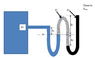# Mulitfluid Manometer

GZunich

## Homework Statement

The manometer shown below measures the pressure of the water in the tank. Using variables for
the densities (no values given), find the gage pressure at point A. Use the symbols ρw, ρ1, and ρ2
for the water and the two manometer fluids.
A picture is attached

## Homework Equations

P1-P2=(g/gc)(densitya(h1-h4)+densityb(h3-h2))

## The Attempt at a Solution

PA=Patm+ρ2(g/gc)(h3+h2)+ρ1(g/gc)(h2)-ρw(g/gc)(h1)
Im not sure if this is correct or not. Because 1 & 2 have increased pressure going down the tube they were added to Patm and the water had less pressure so it was subtracted. I am not sure if this is right so any input would be great.

•